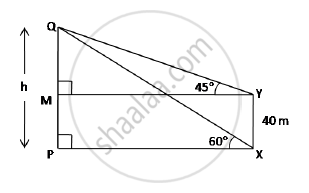# The angle of elevation of the top Q of a vertical tower PQ from a point X on the ground is 60°. From a point Y, 40 m vertically above X, the angle of elevation of the top Q of tower is 45. Find the height of the tower PQ and the distance PX. - Mathematics

The angle of elevation of the top Q of a vertical tower PQ from a point X on the ground is 60°. From a point Y, 40 m vertically above X, the angle of elevation of the top Q of tower is 45. Find the height of the tower PQ and the distance PX. (Use sqrt3=1.73)

#### Solution

MP = YX = 40 m

∴ QM = h - 40

In right angled ΔQMY,

tan45^@=(QM)/(MY)=>1=(h-40)/(PX) " ...."(MY=PX)

∴ PX = h - 40 ....(1)In right angled ΔQPX,

tan60^@=(QP)/(PX)=>sqrt3=(QP)/(PX)

∴ PX=h/sqrt3 " ...(2)"

From (1) and (2), h - 40 = h/sqrt3

:.sqrt(3h)-40sqrt3=h

:.sqrt(3h)-h=40sqrt3

∴ 1.73h - h=40(1.73) ⇒ h = 94.79 m

Thus, PQ is 94.79 m.

Concept: Heights and Distances
Is there an error in this question or solution?